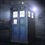# HELP!!!

Yesterday I encountered this problem while I was solving a problem.But don't worry the problem has been solved.Could somebody give a solution to this: $2^{2015}\equiv a(mod{20}).$You have to find 'a'.6 years ago

This discussion board is a place to discuss our Daily Challenges and the math and science related to those challenges. Explanations are more than just a solution — they should explain the steps and thinking strategies that you used to obtain the solution. Comments should further the discussion of math and science.

When posting on Brilliant:

• Use the emojis to react to an explanation, whether you're congratulating a job well done , or just really confused .
• Ask specific questions about the challenge or the steps in somebody's explanation. Well-posed questions can add a lot to the discussion, but posting "I don't understand!" doesn't help anyone.
• Try to contribute something new to the discussion, whether it is an extension, generalization or other idea related to the challenge.

MarkdownAppears as
*italics* or _italics_ italics
**bold** or __bold__ bold
- bulleted- list
• bulleted
• list
1. numbered2. list
1. numbered
2. list
Note: you must add a full line of space before and after lists for them to show up correctly
paragraph 1paragraph 2

paragraph 1

paragraph 2

[example link](https://brilliant.org)example link
> This is a quote
This is a quote
    # I indented these lines
# 4 spaces, and now they show
# up as a code block.

print "hello world"
# I indented these lines
# 4 spaces, and now they show
# up as a code block.

print "hello world"
MathAppears as
Remember to wrap math in $$ ... $$ or $ ... $ to ensure proper formatting.
2 \times 3 $2 \times 3$
2^{34} $2^{34}$
a_{i-1} $a_{i-1}$
\frac{2}{3} $\frac{2}{3}$
\sqrt{2} $\sqrt{2}$
\sum_{i=1}^3 $\sum_{i=1}^3$
\sin \theta $\sin \theta$
\boxed{123} $\boxed{123}$

Sort by:

- 6 years ago

Answer's $8$

- 6 years ago

Yes,I actually know the answer but could you please provide a step-by-step solution?

- 6 years ago

Are you joking? (Really, coz u've solved my problem...)..ANyway...$\phi(20)=8.$ thus, we have that $2^{2015} \pmod {20} = 2^7 \pmod {20}$. Which is nothing but 8!!

- 6 years ago

I think you made a slight mistake as you have applied Euler's Totient theorem which can only be applied when the GCD(a,n)=1.

- 6 years ago

Yes, but it still provides the answer! ^_^. I can't think of any other method.

- 6 years ago

well,thanx for your help but if you can think of any other method please post!!

- 6 years ago

Sure

- 6 years ago

and I think that results are important but the method is more important.

- 6 years ago

You should always have CHINESE REMAINDER THEOREM in mind while solving this type of problems

- 6 years ago

Yes,it was this problem but I figured out that the answer would not be 10 so I solved that problem but, I do not know how to find the remainder.

- 6 years ago

- 6 years ago

2^2015 is a multiple of 4 And 2^2015 leaves a remainder of 3 modulo 5 ( Fermat's little theorem) so, on dividing by 20, I.e.4*5, remainder is 8 as krishna said

Good. Nice solution using CRT. Adarsh Kumar

- 6 years ago

yes awesome!!

- 6 years ago